### Constraints

You can add constraints to a PROC OPTMODEL model. The solver tries to satisfy the specified constraints while minimizing or maximizing the objective.

Constraints in PROC OPTMODEL have names. By using the name, you can examine various attributes of the constraint, such as the dual value that is returned by the solver (see the section Suffixes for details). A constraint is not allowed to have the same name as any other model component. PROC OPTMODEL provides a default name if none is supplied by the constraint declaration. The predefined array `_ACON_` provides names for otherwise anonymous constraints. The predefined numeric parameter `_NACON_` contains the number of such constraints. The constraints are assigned integer indices in sequence, so `_ACON_` refers to the first unnamed constraint declared, while `_ACON_[_NACON_]` refers to the newest.

Consider the following example of a simple model that has a constraint:

```proc optmodel;
var x, y;
min r = x**2 + y**2;
con c: x+y >= 1;
solve;
print x y;
```

Without the constraint named `c`, the solver would find the point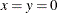that has an objective value of 0. However, the constraint makes this point infeasible. The resulting output is shown in Figure 5.50.

Figure 5.50: Constrained Model Solution

Problem Summary
Objective Sense Minimization
Objective Function r
Objective Type Quadratic

Number of Variables 2
Bounded Above 0
Bounded Below 0
Bounded Below and Above 0
Free 2
Fixed 0

Number of Constraints 1
Linear LE (<=) 0
Linear EQ (=) 0
Linear GE (>=) 1
Linear Range 0

Constraint Coefficients 2

Performance Information
Execution Mode Single-Machine
Number of Threads 4

Solution Summary
Solver QP
Algorithm Interior Point
Objective Function r
Solution Status Optimal
Objective Value 0.4999995397

Primal Infeasibility 2.5005E-7
Dual Infeasibility 2.3570226E-7
Bound Infeasibility 0
Duality Gap 1.9575231E-7
Complementarity 3.9804725E-8

Iterations 3
Presolve Time 0.00
Solution Time 0.01

x y
0.5 0.5

The solver has found the point where the objective function is minimized in the region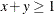. This is actually on the border of the region: the constraint `c` is active (see the section Dual Values for details).

In the preceding example the constraint `c` had only a lower bound. You can specify constraints that have both upper and lower bounds. For example, replacing the constraint `c` in the previous example would further restrict the feasible region:

```   con c: 3 >= x+y >= 1;
```

PROC OPTMODEL standardizes constraints to collect the expression terms that depend on variables and to separate the expression terms that are constant. When there is a single equality or inequality operator, the separable constant terms are moved to the right operand while the variable terms are moved to the left operand. For range constraints, the separable constant terms from the middle expression are subtracted from the lower and upper bounds. You can see the standardized constraints with the use of the EXPAND statement in the following example. Consider the following PROC OPTMODEL statements:

```proc optmodel;
var x{1..3};
con b: sum{i in 1..3}(x[i] - i) = 0;
expand b;
```

These statements produce an optimization problem with the following constraint:

```   (x - 1) + (x - 2) + (x - 3) = 0
```

The EXPAND statement produces the output in Figure 5.51.

Figure 5.51: Expansion of a Standardized Constraint

 ```Constraint b: x + x + x = 6 ```

Here the `i` separable constant terms in the operand of the SUM operation were moved to the right-hand side of the constraint. The sum of these `i` values is 6.

After standardization the constraint expression that contains all the variables is called the body of the constraint. You can reference the current value of the body expression by attaching the `.body` suffix to the constraint name. Similarly, the upper and lower bound expressions can be referenced by using the `.ub` and `.lb` suffixes, respectively. (See the section Suffixes for more information.)

As a result of standardization, the value of a body expression depends on how the corresponding constraint is entered. The following example demonstrates how using equivalent relational syntax can result in different `.body` values:

```proc optmodel;
var x init 1;
con c1: x**2 <= 5;
con c2: 5 >= x**2;
con c3: -x**2 >= -5;
con c4: -5 <= -x**2;
expand;
print c1.body c2.body c3.body c4.body;
```

The EXPAND and PRINT statements produce the output in Figure 5.52.

Figure 5.52: Expansion and Body Values of Standardized Constraints

 ```Var x Constraint c1: x**2 <= 5 Constraint c2: -x**2 >= -5 Constraint c3: -x**2 >= -5 Constraint c4: --x**2 <= 5 ```

c1.BODY c2.BODY c3.BODY c4.BODY
1 -1 -1 1

Caution: Each constraint has an associated dual value (see Dual Values). As a result of standardization, the sign of a dual value depends in some instances on the way in which the corresponding constraint is entered into PROC OPTMODEL. In the case of a minimization objective with one-sided constraint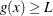, avoid entering the constraint as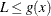. For example, the following statements produce a value of 2:

```   proc optmodel;
var x;
min o1 = x**2;
con c1: x >= 1;
solve;
print (c1.dual);
```

Replacing the constraint as follows results in a value of –2:

```      con c1: 1 <= x;
```

In the case of a maximization objective with the one-sided constraint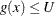, avoid entering the constraint as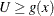.

When a constraint has variables on both sides, the sign of the dual value depends on the direction of the inequality. For example, you can enter the following constraint:

```      con c1: x**5 - y + 8 <= 5*x + y**2;
```

This is a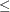constraint, so `c1.dual` is nonpositive. If you enter the same constraint as follows, then `c1.dual` is nonnegative:

```      con c1: 5*x + y**2 >= x**5 - y + 8;
```

It is also important to note that the signs of the dual values are negated in the case of maximization. The following statements output a value of 2:

```   proc optmodel;
var x;
min o1 = x**2;
con c1: 1 <= x <= 2;
solve;
print (c1.dual);
```

Changing the objective function as follows yields the same value of `x`, but `c1.dual` now holds the value –2:

```      max o1 = -x**2;
```

Note: A simple bound constraint on a decision variablecan be entered either by using a CONSTRAINT declaration or by assigning values to `x.lb` and `x.ub`. If you require dual values for simple bound constraints, use the CONSTRAINT declaration.

Constraints can be linear or nonlinear. PROC OPTMODEL determines the type of constraint automatically by examining the form of the body expression. Subexpressions that do not involve variables are treated as constants. Constant subexpressions that are multiplied by or added to linear subexpressions produce new linear subexpressions. For example, constraint `A` in the following statements is linear:

```   proc optmodel;
var x{1..3};
con A: 0.5*(x-x) + x >= 0;
```